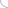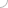# True discount - aptitude test

Home > Aptitude test > True discount

### 1) If the true discount on Rs.4510 due 6 months hence is Rs.110, the rate of interest is:

a) 5%
b) 10%
c) 4%
d) 8%

Solution :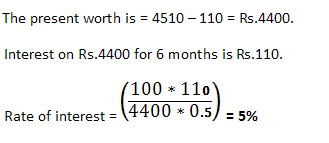### 2) If simple interest and true discount on a certain amount for the same time period and same rate of interest is Rs.130 and Rs.120 respectively, then find the value of the amount.

a) 1300
b) 1200
c) 1560
d) 1440

Solution :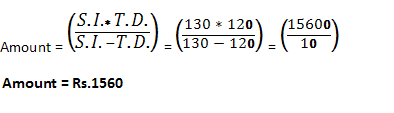### 3) A man owes a lender Rs.20502 due 1 year hence. If the man wants to settle the account after 8 months and the rate of interest being 6% per annum, find the amount the man should pay to the lender.

a) 20000
b) 20100
c) 20200
d) None of these

Solution :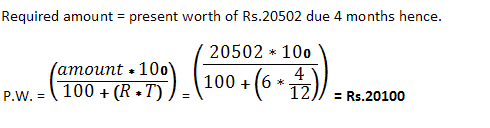### 4) A man purchased a bicycle for Rs.4800 and sold it immediately at Rs.5995, allowing the buyer a credit of one and a half years. If the rate of interest is 6%, then the man gains:

a) Rs.700
b) Rs.1195
c) Rs.495
d) Rs.900

Solution :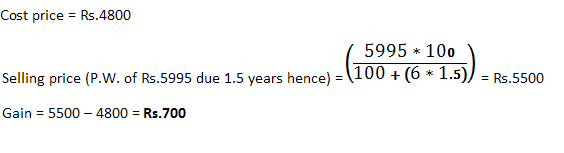### 5) Gopal has to pay Rs.440 to Ajay after 1 year. Ajay asks Gopal to pay Rs.220 in cash and defer the payment of Rs.220 for 2 years. If the rate of interest is 10% per annum, in this mode of payment:

a) Gopal gains Rs.3.33
b) Ajay gains Rs.3.33
c) Ajay loses Rs.16.67
d) Gopal loses Rs.16.67

Solution :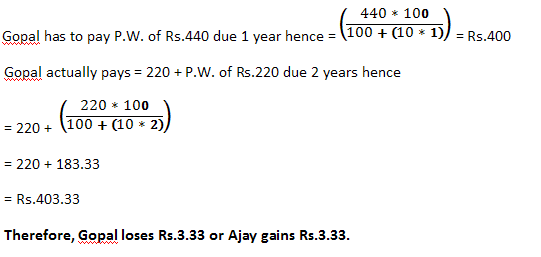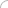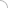Aptitude questions